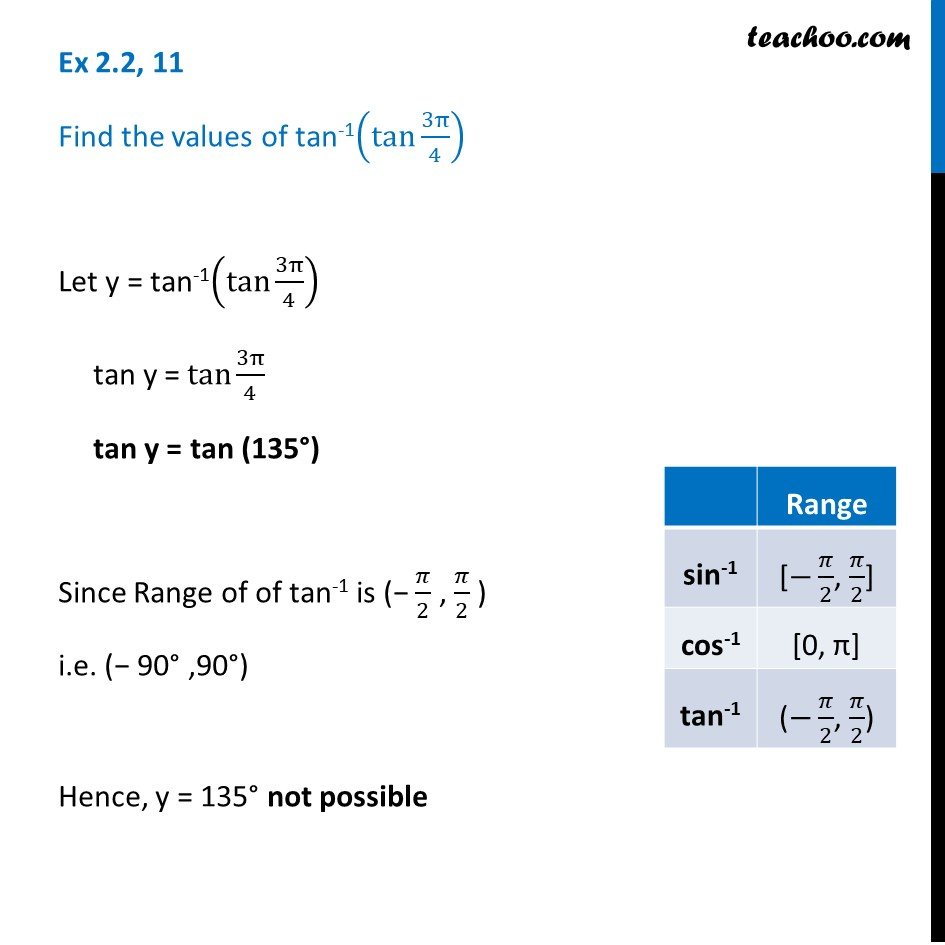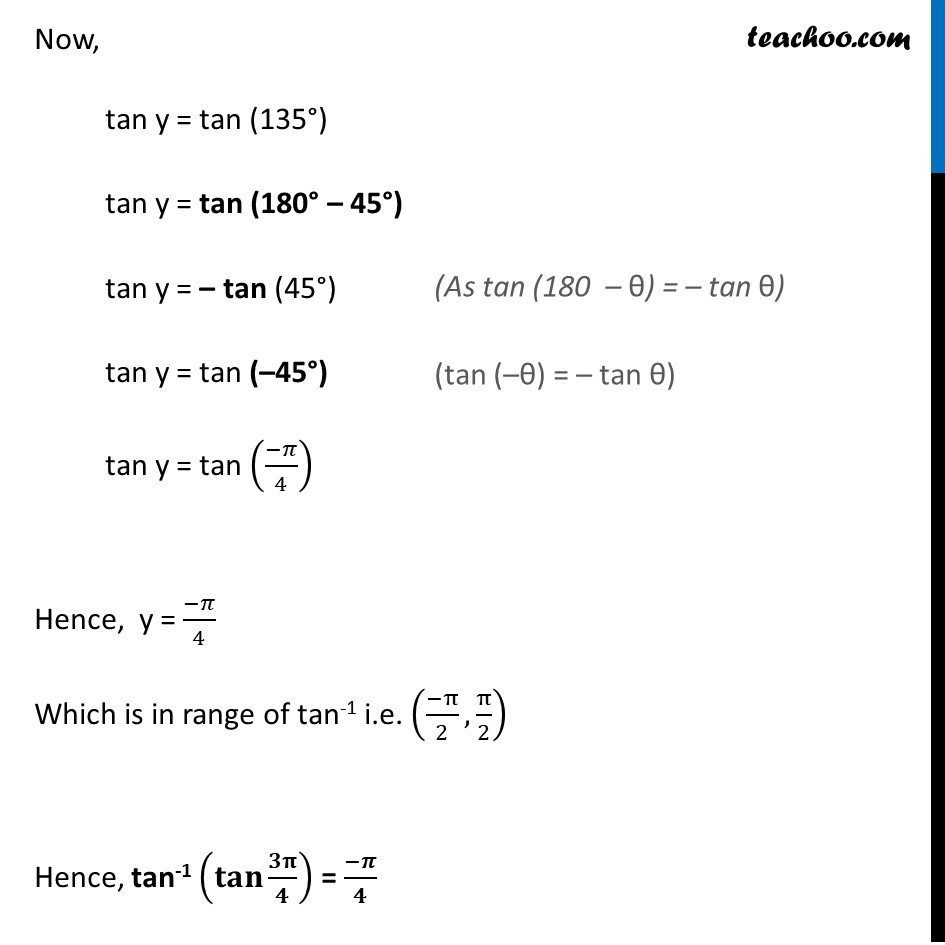Ex 2.2

Chapter 2 Class 12 Inverse Trigonometric Functions
Serial order wiseLearn in your speed, with individual attention - Teachoo Maths 1-on-1 Class

### Transcript

Ex 2.2, 11 Find the values of tan-1(tan⁡〖3π/4〗 ) Let y = tan-1(tan⁡〖3π/4〗 ) tan y =〖 tan〗⁡〖3π/4〗 tan y = tan (135°) Since Range of of tan-1 is (− 𝜋/2 , 𝜋/2 ) i.e. (− 90° ,90°) Hence, y = 135° not possible Now, tan y = tan (135°) tan y = tan (180° – 45°) tan y = – tan (45°) tan y = tan (–45°) tan y = tan ((−𝜋)/4) Hence, y = (−𝜋)/4 Which is in range of tan-1 i.e. ((−π)/2, π/2) Hence, tan-1 (𝐭𝐚𝐧⁡〖𝟑𝛑/𝟒〗 ) = (−𝝅)/𝟒